## Video

```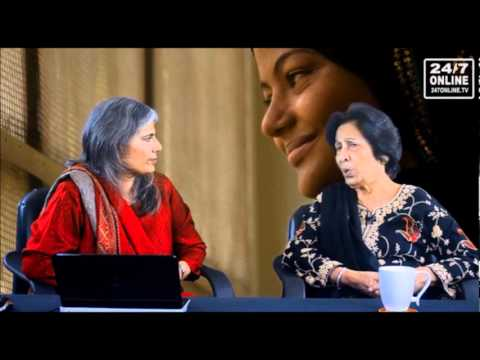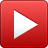/* <![CDATA[ */
\$(function(){
var width = \$(window).innerWidth();
var setwidth = 640;
var ratio = 0.75;
var height = 480;
if(width < setwidth)
{
height = Math.floor(width * 0.75);
//console.log("device width "+width+", set width "+640+", ratio "+0.75+", new height "+ height);
var new_url = wpvl_paramReplace('width', link, width);
var new_url = wpvl_paramReplace('height', new_url, height);
\$("a#62efe5ee4aa56").attr('href', new_url);
//console.log(new_url);
}
});
});
/* ]]&gt; */
```
Justice Majida (R) Razvi interviews (Part 1)
```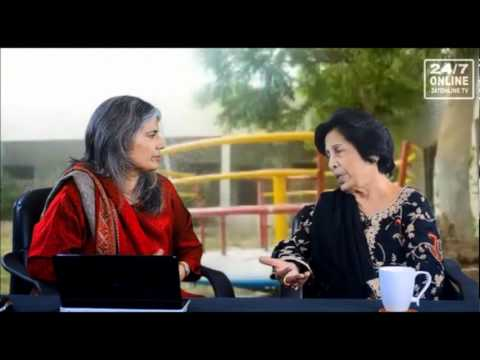/* <![CDATA[ */
\$(function(){
var width = \$(window).innerWidth();
var setwidth = 640;
var ratio = 0.75;
var height = 480;
if(width < setwidth)
{
height = Math.floor(width * 0.75);
//console.log("device width "+width+", set width "+640+", ratio "+0.75+", new height "+ height);
var new_url = wpvl_paramReplace('width', link, width);
var new_url = wpvl_paramReplace('height', new_url, height);
\$("a#62efe5ee4ae77").attr('href', new_url);
//console.log(new_url);
}
});
});
/* ]]&gt; */
```
Justice Majida (R) Razvi interviews (Part 2)
```/* <![CDATA[ */
\$(function(){
var width = \$(window).innerWidth();
var setwidth = 640;
var ratio = 0.75;
var height = 480;
if(width < setwidth)
{
height = Math.floor(width * 0.75);
//console.log("device width "+width+", set width "+640+", ratio "+0.75+", new height "+ height);
var new_url = wpvl_paramReplace('width', link, width);
var new_url = wpvl_paramReplace('height', new_url, height);
\$("a#62efe5ee4b27d").attr('href', new_url);
//console.log(new_url);
}
});
});
/* ]]&gt; */
```
```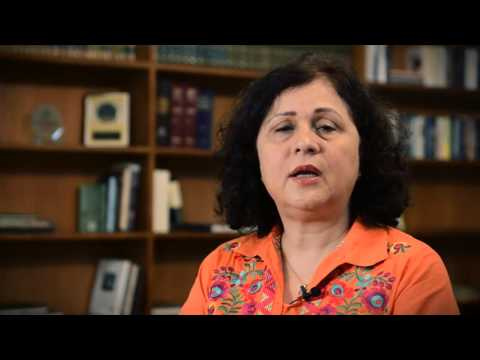/* <![CDATA[ */
\$(function(){
var width = \$(window).innerWidth();
var setwidth = 640;
var ratio = 0.75;
var height = 480;
if(width < setwidth)
{
height = Math.floor(width * 0.75);
//console.log("device width "+width+", set width "+640+", ratio "+0.75+", new height "+ height);
var new_url = wpvl_paramReplace('width', link, width);
var new_url = wpvl_paramReplace('height', new_url, height);
\$("a#62efe5ee4b875").attr('href', new_url);
//console.log(new_url);
}
});
});
/* ]]&gt; */
```
Uzma Noorani, an alumna of the State Department’s International Visitor Leadership Program
```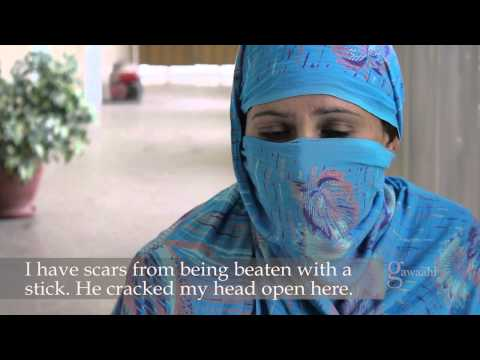/* <![CDATA[ */
\$(function(){
var width = \$(window).innerWidth();
var setwidth = 640;
var ratio = 0.75;
var height = 480;
if(width < setwidth)
{
height = Math.floor(width * 0.75);
//console.log("device width "+width+", set width "+640+", ratio "+0.75+", new height "+ height);
var new_url = wpvl_paramReplace('width', link, width);
var new_url = wpvl_paramReplace('height', new_url, height);
\$("a#62efe5ee4bc76").attr('href', new_url);
//console.log(new_url);
}
});
});
/* ]]&gt; */
```
Gawaahi – A survivor’s story by Amina Agha
```/* <![CDATA[ */
\$(function(){
var width = \$(window).innerWidth();
var setwidth = 640;
var ratio = 0.75;
var height = 480;
if(width < setwidth)
{
height = Math.floor(width * 0.75);
//console.log("device width "+width+", set width "+640+", ratio "+0.75+", new height "+ height);
var new_url = wpvl_paramReplace('width', link, width);
var new_url = wpvl_paramReplace('height', new_url, height);
\$("a#62efe5ee4c070").attr('href', new_url);
//console.log(new_url);
}
});
});
/* ]]&gt; */
```